# 1. 直观解释e

###Looking at e’s history, it seems it has something to do with growth or interest rates. e was discovered when performing business calculations (not abstract mathematical conjectures) so “interest” (growth) is a possible theme.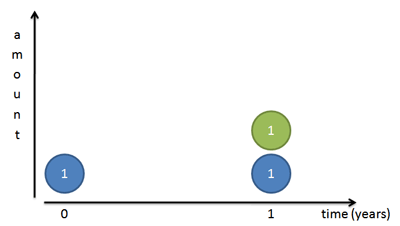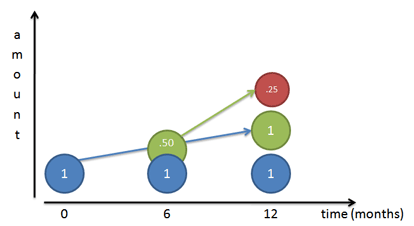• 每12个月：growth = (1 + 1)
• 每6个月：growth = (1 + 1/2)^2
• 每3个月：growth = (1 + 1/3)^3
• 结算周期无穷算（e is defined to be that rate of growth if we continually compound 100% return on smaller and smaller time periods）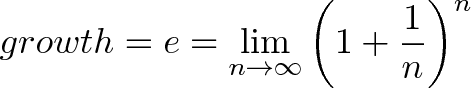n          (1 + 1/n)^n
-----------------------
1          2
2          2.25
3          2.37
5          2.488
10         2.5937
100        2.7048
1,000      2.7169
10,000     2.71814
100,000    2.718268
1,000,000  2.7182804

e is about 100% continuous growth.

e is the base rate of growth shared by all continually growing processes. e lets you take a simple growth rate (where all change happens at the end of the year) and find the impact of compound, continuous growth, where every nanosecond (or faster) you are growing just a little bit.

e represents the idea that all continually growing systems are scaled versions of a common rate.

Just like every number can be considered a scaled version of 1 (the base unit), every circle can be considered a scaled version of the unit circle (radius 1), and every rate of growth can be considered a scaled version of e (unit growth, perfectly compounded).

## 1.1 形式化定义直观解释

possible）

• 1 = 1/0!，本金
• 1 = 1/1!，由本金直接产生的利息
• 0.5 = 1/2!，由利息产生的利息，第二级
• 0.1666 = 1/3!，由利息产生的利息，第三级

• 需要1个单位时间从1增加到2
• 需要1/2个单位时间从2增加到3
• 需要1/3个单位时间从3增加到4

ln(a)表示从1增加到a需要的时间。ln(e)=1可以视为花一个单位时间所能达到的增长量（is is the amount of growth after waiting exactly 1 unit of time）。

Define e as always growing by 100% of your current value

# 2. 自然对数

Define the time needed to grow continuously from 1 to as ln(a).

# 3. 指数函数

（1）不同的利率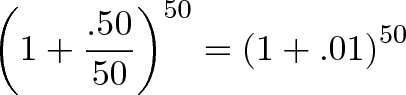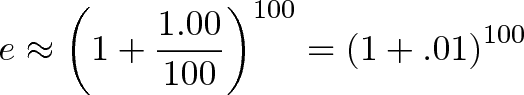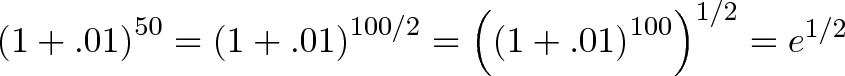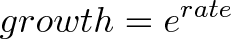（2）不同的时长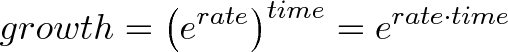• x is the number of times we multiply a growth rate: 100% growth for 3 years is e3
• x is the growth rate itself: 300% growth for one year is e3

BetterExplained: An Intuitive Guide To Exponential Functions & e
Math, BetterExplained.pdf
Wikipedia: e (mathematical constant)
知乎：数学里的 e 为什么叫做自然底数？是不是自然界里什么东西恰好是 e？
BetterExplained: A Visual Guide to Simple, Compound and Continuous Interest Rates微信赞赏支付宝赞赏